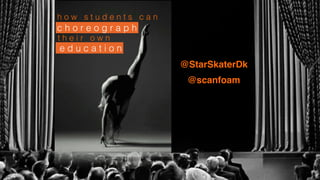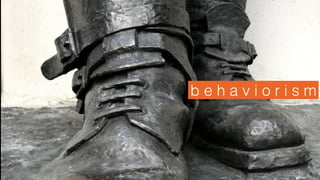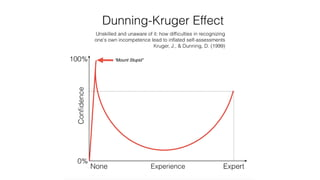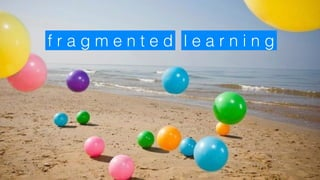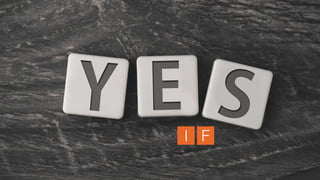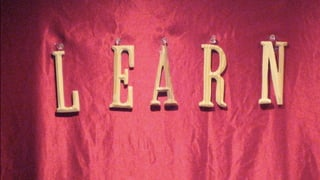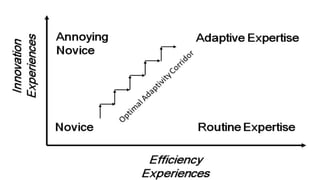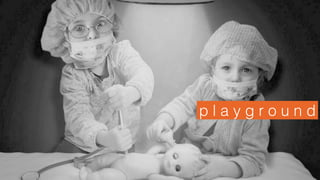1 of 31

### The Art of Learning Medicine: Sandra Viggers

1. @StarSkaterDk @scanfoam c h o r e o g r a p h e d u c a t i o n h o w s t u d e n t s c a n t h e i r o w n
2. c o n f e s s i o n t i m e
3. No!
4. b e h a v i o r i s m
5. l a b. d o g M D
6. h u m a n i s m
7. p o t e n t i a l
8. m o t i v a t i o n
9. l e a r n i n gf r a g m e n t e d
10. c o n s t r u c t i v i s m
11. I F
12. c o n n e c t i v i s m
13. c h a l l e n g e r i s k f r e e r e f l e c t
14. p l a y g r o u n d
15. t h e “g y m “
16. r e p e t i t i o n s p a c e d
17. e d u c a t i o n t a k e s t i m e
18. r e f l e c t i o n
19. e x c e l l e n c e